Definitions of Square Dance Calls and Concepts
Trapezoid Formation [C3B]

Index -->  Plus  |  A1  |  A2  |  C1  |  C2  |  C3A  |  C3B  |  C4  |  NOL  |
Definitions (Text Only) -->  Plus  |  A1  |  A2  |  C1  |  C2  |  C3A  |  C3B  |  C4  |  NOL  |
 Find call:

Trapezoid Formation -- [C3B]
(author unknown)

 \$B8@8l(BA Trapezoid is a Distorted 2 x 2 that can be formed from a normal 2 x 2 after any two adjacent dancers each move a distance of one matrix position directly away from each other.  EN: 10
Trapezoid (\$BBf7A(B) \$B\$O(B Distorted (\$B\$f\$,\$s\$@(B) 2 x 2 \$B\$G(B, \$BIaDL\$N(B 2 x 2 \$B\$+\$iNY\$j9g\$C\$??M\$N\$I\$A\$i\$+\$,(B, \$B\$*8_\$\$\$KN%\$l\$k\$h\$&\$K(B 1 \$B%]%8%7%g%sF0\$\$\$?\$H\$-\$K\$G\$-\$^\$9(B. JP: 10

From a 2 x 4, there are two Trapezoids. The Ends of one side work with the Centers of the other side within a Distorted Box. EN: 20
2 x 4 \$B\$+\$i(B, 2 \$B\$D\$N(B Trapezoid \$B\$,\$"\$j\$^\$9(B. Ends \$B\$O(B, \$BH?BPB&\$N(B Centers \$B\$H(B Distorted Box \$B\$GF0\$-\$^\$9(B. JP: 20

Trapezoid Formation: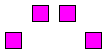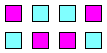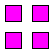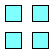From here... EN: 30 \$B\$3\$3\$+\$i(B... JP: 30 imagine you are here... EN: 40 \$B\$3\$3\$K\$\$\$k\$H;W\$C\$F(B... JP: 40 or here. EN: 50 \$B\$^\$?\$O\$3\$3(B. JP: 50

\$BCm
• The traffic pattern for some Trapezoid calls can be horrendous. Use Stars as necessary and try to get to your ending position without colliding with any other dancers. EN: 60
\$B\$"\$k(B Trapezoid \$B\$N%3!<%k\$NDL\$jJ}\$O(B, \$B\$H\$s\$G\$b\$J\$\$\$b\$N\$K\$J\$j\$^\$9(B. \$BI,MW\$J\$i(B Star \$B\$r;H\$C\$F(B, \$BB>\$N?M\$H\$V\$D\$+\$i\$J\$\$\$h\$&\$K\$7\$F(B, \$B<+J,\$N=*\$o\$k>l=j\$X9T\$/\$h\$&\$K\$7\$F\$/\$@\$5\$\$(B. JP: 60
• Calls must end on original Trapezoid footprints. Shape-changing calls are not allowed. EN: 70
\$B%3!<%k\$G\$O(B, \$B%*%j%8%J%k\$N(B Trapezoid \$B\$N>l=j\$G=*\$o\$kI,MW\$,\$"\$j\$^\$9(B. Shape change \$B\$N%3!<%k\$O(B, \$B5v\$5\$l\$^\$;\$s(B. JP: 70
• Trapezoid Couple Up: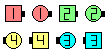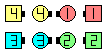Trapezoid Couple Up\$B\$NA0(B \$B8e(B

Once Removed Trapezoid Counter Rotate 1/4:
(This is very difficult, even at C4) EN: 80
(\$B\$3\$l\$O(B, C4 \$B\$G\$"\$C\$F\$b(B, \$B\$H\$F\$bFq\$7\$\$\$G\$9(B) JP: 80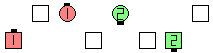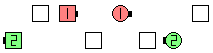Once Removed TrapezoidCounter Rotate 1/4\$B\$NA0(B \$B8e(B

\$BMagic Line | Wave CONCEPT [C3B]

which (from Parallel Lines) involves the same footprints, but dancers work within a Distorted Line instead of a Distorted Box. EN: 90
Magic \$B\$G\$O(B, \$BJ?9T\$J(B Line \$B\$+\$iF1\$80LCV\$GF0\$-\$^\$9\$,(B, Distorted Box \$B\$G\$J\$/(B Distorted Line \$B\$GF0\$-\$^\$9(B. JP: 90Choreography for Trapezoid FormationComments? Questions? Suggestions?

https://www.ceder.net/def/trapezoid.php?language=japan&level=C3B&action=edit
11-December-2019 20:25:02